# Imagine

Imagine that a unit of air at a temperature of 25°C rises up a mountain range that is 3,000 meters high on the windward side and which descends to 1,200 meters on the leeward side, assuming that the air will remain dry what will its temperature when it crosses the top of the range and what will it be when it descends to the base on the lee side? (temperature decreases 6 degrees Celcius for every 1000 meter)

t1 =  7 °C
t2 =  17.8 °C

### Step-by-step explanation: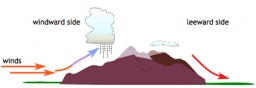Did you find an error or inaccuracy? Feel free to write us. Thank you!## Related math problems and questions:

• Air thermal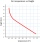Imagine that a unit of air rises at 3000 meters high, if temperature decreases 6 degrees celcius for every 1000 meter, what will be its temperature at 1400 meters, 2000 meters, 2500 meters and when it reaches the 3000 meter elevation. Starting temperature
• Electrics - conductor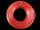The wire is 106 meters long at 0 °C and at every temperature increase of 1 °C the length increases by 0.15 mm per 1 m length of wire. Determine a function which represents the overall length of the wire as a function of temperature. What is the length of
• Latent heat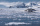How much heat is needed to take from 100 g of water at 20°C to cool to ice at -18°C? Mass heat capacity c (ice) = 21kJ/kg / °C; c (water) = 4.19 KJ/kg / °C and the mass group heat of solidification of water is l = 334kJ/kg
• WeatherWeather. At 5:00 am, the temperature was 28°C, and at noontime, it rose by 9°C. By 4:00 pm, it dropped 3°C, and by nighttime, it was 4°C less. What is the room temperature this time?
• Now isNow is 5°C morning. What will the temperature be if i. It rises by 12°C ii. It falls by 15°C
• Water mixingWe have 520 ml of hot water and 640 ml of water at 48°C. What is the temperature of approximately hot water when the resulting mixture has a temperature of 65°C?
• The bathDad poured 50 liters of water at 60°C into the tub. How much must cold water be added at 10°C to obtain a bath that has a temperature of 40°C?
• Water temperaturesTo 25 liters of water at 50 °C we add 15 liters of water at a different temperature. How many °C should the water be colder than 50 °C so that 40 liters of the water obtained has a temperature 42.5 °C?
• The poolThe pool has a volume of 40 m3, and the water temperature is 20 °C. How much water at 100 °C should we pour into the pool to increase the water temperature by 5 °C?
• The bathThe bath has water with a volume of 220 l and a temperature of 65°C. Determine the mass m2 of water at 15°C to be poured so that the resulting temperature of the water in the bath is 40°C. We neglect the heat exchange between water and the environment. T
• Compressed gas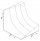The pressure vessel contains a compressed gas at a temperature t1 = 27°C and a pressure p1 = 4 MPa. How much does its pressure change when we release half the amount of gas and its temperature drops to t2 = 15°C?
• ThereThere is 5 l of water with a temperature of 20°C in the bucket. We add 3 liters of hot water. The resulting temperature will be 30°C. What was the temperature of the hot water?
• Mixing waterWe should fill the 30-liter container with water at 60 degrees Celsius. How many liters of water 80 degrees C hot, and how many liters of water 20 degrees Celsius warm we have to mix?
• The aluminumThe aluminum object with a weight of 0.80 kg and a temperature of 250°C was placed in the water with a weight of 1.6 kg and a temperature of 23°C. What is the system temperature after reaching equilibrium? We assume that the heat exchange occurred only be
• Thermometer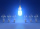Immerse a thermometer with a heat capacity of 2.0 J. K-1 in water weighing 67.0 grams. Before immersion in water, the thermometer showed a temperature of 17.8 degrees Celsius. After reaching equilibrium, the temperature is 32.4 degrees. What was the water
• The temp rangeWhat is the range in temperatures if the high temperatures is 67 degrees Celsius and the low temperature is -25 degrees Celsius?
• Mine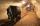What is temperature in the mine at a depth of 1160 m, where at depth 9 m is 11°C and every 100 m, the temperature increases by 0.7°C?